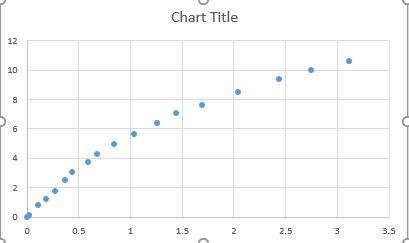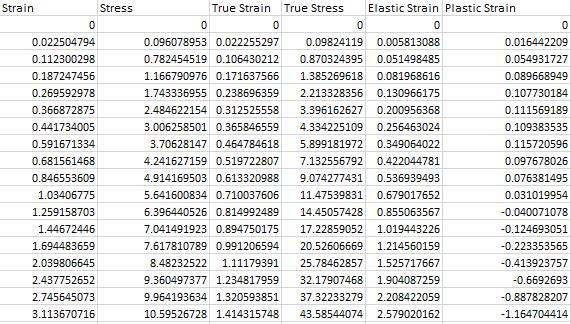# Entering Material Model for a Polymer using Multilinear hardening model

Hello

I am trying to use the plastic strain failure model for a material with the below stress-strain curve.I use the relation introduced in this thread (https://studentcommunity.ansys.com/thread/multilinear-kinematic-plasticity-material-model-create-from-a-stress-strain-graph/) to obtain true stress-true strain curve and "plastic strain". But the problem is that large value of strain in this polymeric material leads to a negative value for plastic strain. The below table:I wonder how I can enter this data for my material model in a multilinear hardening? And if I use Plastic Strain Failure criteria for failure, what value I should set for maximum EPS?

(I have attached the excel datasheet to this thread).

Hamed

• Are the values of strain in terms of % strain, so they go over 3% strain?

Or are the values of strain in terms of mm/mm and so they go over 300% strain?

The equations to convert stress-strain data to True Stress and True Strain are not valid after the part starts necking or has other localized failure conditions.

• The strains are in mm/mm and yes, they go over 300%.

I took the data from previous works and have not seen the sample but I don't think it goes through necking. The material is DuPont Surlyn Ionomer, and it is quite stretchable. So in this case what material model and failure model should I use to predict failure correctly?

• Use a Hyperelasticity material model. There are many to choose from in the Engineering Data tab of Workbench. These models are constructed by fitting model coefficients to experimental data, often from multiple types of experiments like uniaxial tension, biaxial tension, uniaxial compression, etc.  You only have uniaxial tensile data. It helps if you have data from more than one type of test.

The Engineering Data has Hyperelastic Curve Fitting programs that take your data and calculate a best fit to the model coefficients.  Read more about this in the ANSYS Help system under Mechanical APDL > Material Reference and watch some YouTube videos.  Try a few different simpler models like Mooney-Rivelin, Ogden, or Yeoh, and use the smallest number of coefficients first.

Hyperelastic materials do not exhibit plasticity, which is for ductile metals.

I have seen Ultimate Tensile Strength for hyperelastic materials that would be an appropriate tensile failure criteria, but there are other failure mechanisms for a design such as compression set over long periods of time.

• Thanks for your answer. As you mentioned the main problem with Hyperelastic materials is that they do not exhibit plasticity but the material that I am using becomes fully plastic in large deformation that's why I used multilinear hardening model. So I wonder in this case and by having only the engineering stress-strain curves (as shown above) what kind of material model can be utilized?

• So Peter! With your answer should I conclude we cannot model this material in ansys although we the engineering stress-strain curve?

• There are elastomers that are elastic out to 300% with no permanent deformation. That is what the Hyperelasticity material models are for.

You have not provided any data to differentiate where plasticity occurs! There is not plasticity at the beginning, right? Even when it begins, some of the stretch will be elastic while some will be plastic. The stress-strain curve alone is not sufficient because both elastic and plastic deformation are blended into the one number.

• I don't have the exact information about when plasticity starts but approximately and through the experiments that I have conducted, I can say after 100% of elongation, the plasticity begins. So what other information is necessary to model this? Should I combine a Hyperelasticity model with Plastic Failure? If yes, how should I calculate the maximum EPS?

• I conducted further experiments and now I can say that plasticity begins at engineering stress of 6.39MPa and strain of 1.26 (which is equivalent to true stress and strain of 14.5 MPa and 0.82). Knowing this information, what value should I use for maximum EPS?

• I have not tried to combine hyperelasticity and plasticity but the ANSYS Engineering Data allows some combinations. You will have to try them out and also use the hyperelastic curve fitting program to extract model constants from your stress-strain data.